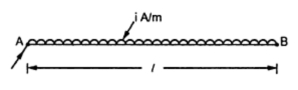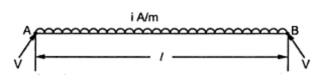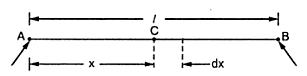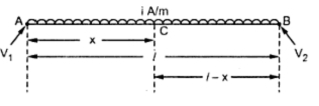### D.C. Distributor With Distributed Load

This type of distributor is also classified as,
1. Distributor fed at one end
2. Distributor fed at both the ends
1.1 Distributor Load Fed at One End
The Fig. 1 shows the single line diagram of uniformly distributed load on two wire distributor, fed at one end.Fig. 1

Th Distributor load is of i amperes per meter.
This indicates that at every metre length i amperes load is supplied through tapping on the distributor.
Let      l  = Length of the distributor
r = Resistance per unit length in Ω
In such case, total voltage drop is to be obtained by considering a point C at a distance x from feeding end A.
This is shown in the Fig. 2.Fig. 2

The current tapped at point C is
= Total current - Current up to point C
= i x  l  - i x x
= i (- x)
Consider an elementary length dx near point C. Its resistance is r dx.
Hence the voltage drop over the length dx is
dV = i (- x) r dx
Thus total voltage drop upto point C is,

This is the equation of parabola.
Thus the voltage drop upto point B can be calculated by putting x =  l .

where        I = Total current fed at point A
R = Total resistance of the distributor
From this one important observation can be noted as :
In a uniformly distributed load on the distributor fed at one end, the total voltage drop is equal to that produced by the whole of the load assumed to be concentrated at the middle point. This fact can be used to simplify the complicated load calculations.Fig. 3  Current loading and voltage drop diagram

The power loss i.e. I2R loss can be obtained from the elementary length dx.
The current at any point C at distance x is i (l -x). Hence the power loss over the elementary length dx is,

1.2 Distributed Load Fed at Both Ends
This is type is further classified as,
1. Ends maintained at equal voltages.
2. Ends maintained at unequal voltages.
1.2.1 Ends at Equal Voltages
The Fig. 4 shows a distributor of length l  with uniform load of i amperes per meter. The resistance of the conductor is r ohms per metre. It is fed at the points A and B which are maintained at equal voltages.Fig. 4
The total current to be supplied is i amperes.
As two end voltages are equal. each end will supply half the required current i.e. i /2.
The point of minimum potential will be at a distance of /2 from either end i.e. midpoint.
Consider a point C at a distance x metres from feeding end A as shown in the Fig. 5.Fig. 5

The current entering at feeding end A is (i /2).
Hence the current at point C can be written as,

Consider an elementary length dx near C whose resistance is rdx.
So voltage drop over length dx is,

Hence voltage drop upto point C is,

Now maximum voltage drop is at midpoint i.e. x = /2 as the midpoint is the point of minimum potential.

where I is total current and R is the total resistance.
It is 1/4 th of the drop in case of distributed load distributor fed at one end. The point of minimum potential is the farthest end from feeding end in case of distributor fed at one end while in this case, it is midpoint of the length of the distributor.Fig. 6  Current loading and voltage drop diagram

The power loss in this case also can be obtained from the power loss over elementary length dx.

This is power loss over the entire length l  of the distributor. Hence power loss in half length is half of the total power loss which is (i2)/24 watts.
1.2.2  Ends at Unequal Voltages
The Fig. 7 shows the uniformly distributed load on the distributor of length  . The load is i amperes/m while the distributor is fed at both the ends which are maintained at different voltages.Fig. 7

To find the location of point of minimum potential :
Let point C be the point of minimum potential which is at a distance x from feeding point A.
The current supplied by the feeding point A is ix. While the current supplied by the feeding point B is i ( - x).
Now, V1 - drop in section AC = V2 - drop in section BC.
Let r be the resistance per metre length.
In case of distributed load the drop is given by ( i  2/2 for a length of ' '. Considering section AC as separate section fed at one end the drop in section AC can be written as,

Knowing V1V2, i, r and  , the above equation can be solved for x which gives the point of minimum potential.

1.Love to read from start to end … you have good information I really like that also your work is appreciative thank you for sharing it with us J

www.schnap.com.au

2.very nice good work

3.thank you very much